GeeksforGeeks App
Open AppBrowser
Continue

# Delete a node in a Doubly Linked List

Write a function to delete a given node in a doubly-linked list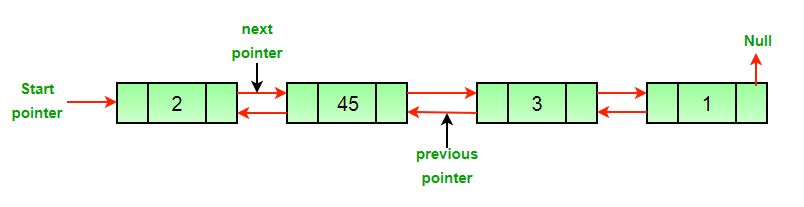Example:

Input: DLL = 2->45->3->1, Node = 45
Output:  2->3->1

Input: DLL = 2->45->3->1, Node = 1
Output:  2->45->3

Approach: The deletion of a node in a doubly-linked list can be divided into three main categories:

• After the deletion of the head node.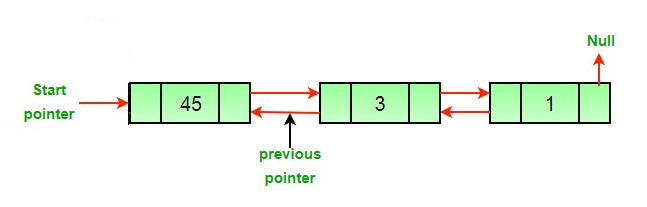• After the deletion of the middle node.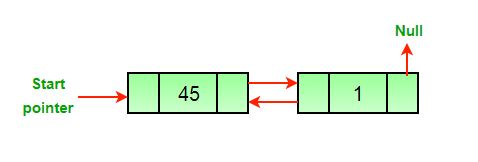• After the deletion of the last node.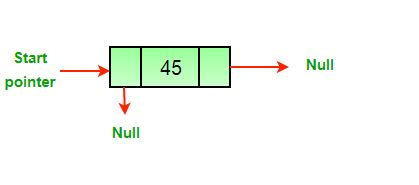All three mentioned cases can be handled in two steps if the pointer of the node to be deleted and the head pointer is known.

1. If the node to be deleted is the head node then make the next node as head.
2. If a node is deleted, connect the next and previous node of the deleted node.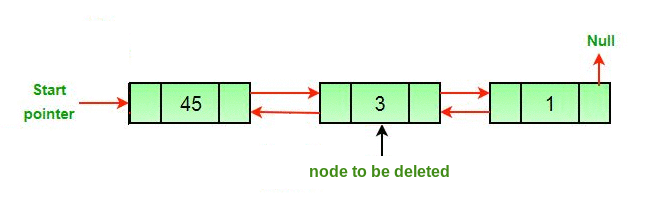Algorithm:

• Let the node to be deleted be del.
• If node to be deleted is head node, then change the head pointer to next current head.
```if headnode == del then
• Set prev of next to del, if next to del exists.
```if del.nextNode != none
del.nextNode.previousNode = del.previousNode ```
• Set next of previous to del, if previous to del exists.
```if del.previousNode != none
del.previousNode.nextNode = del.next```

Below is the implementation of the above approach:

## C++

 `// C++ program to delete a node from``// Doubly Linked List``#include ``using` `namespace` `std;`` ` `/* a node of the doubly linked list */``class` `Node ``{ ``    ``public``:``    ``int` `data; ``    ``Node* next; ``    ``Node* prev; ``}; `` ` `/* Function to delete a node in a Doubly Linked List. ``head_ref --> pointer to head node pointer. ``del --> pointer to node to be deleted. */``void` `deleteNode(Node** head_ref, Node* del) ``{ ``    ``/* base case */``    ``if` `(*head_ref == NULL || del == NULL) ``        ``return``; `` ` `    ``/* If node to be deleted is head node */``    ``if` `(*head_ref == del) ``        ``*head_ref = del->next; `` ` `    ``/* Change next only if node to be ``    ``deleted is NOT the last node */``    ``if` `(del->next != NULL) ``        ``del->next->prev = del->prev; `` ` `    ``/* Change prev only if node to be ``    ``deleted is NOT the first node */``    ``if` `(del->prev != NULL) ``        ``del->prev->next = del->next; `` ` `    ``/* Finally, free the memory occupied by del*/``    ``free``(del); ``    ``return``; ``} `` ` `/* UTILITY FUNCTIONS */``/* Function to insert a node at the``beginning of the Doubly Linked List */``void` `push(Node** head_ref, ``int` `new_data) ``{ ``    ``/* allocate node */``    ``Node* new_node = ``new` `Node();`` ` `    ``/* put in the data */``    ``new_node->data = new_data; `` ` `    ``/* since we are adding at the beginning, ``    ``prev is always NULL */``    ``new_node->prev = NULL; `` ` `    ``/* link the old list of the new node */``    ``new_node->next = (*head_ref); `` ` `    ``/* change prev of head node to new node */``    ``if` `((*head_ref) != NULL) ``        ``(*head_ref)->prev = new_node; `` ` `    ``/* move the head to point to the new node */``    ``(*head_ref) = new_node; ``} `` ` `/* Function to print nodes in a given doubly linked list ``This function is same as printList() of singly linked list */``void` `printList(Node* node) ``{ ``    ``while` `(node != NULL) ``    ``{ ``        ``cout << node->data << ``" "``; ``        ``node = node->next; ``    ``} ``} `` ` `/* Driver code*/``int` `main() ``{ ``    ``/* Start with the empty list */``    ``Node* head = NULL; `` ` `    ``/* Let us create the doubly linked list 10<->8<->4<->2 */``    ``push(&head, 2); ``    ``push(&head, 4); ``    ``push(&head, 8); ``    ``push(&head, 10); `` ` `    ``cout << ``"Original Linked list "``; ``    ``printList(head); `` ` `    ``/* delete nodes from the doubly linked list */``    ``deleteNode(&head, head); ``/*delete first node*/``    ``deleteNode(&head, head->next); ``/*delete middle node*/``    ``deleteNode(&head, head->next); ``/*delete last node*/`` ` `    ``/* Modified linked list will be NULL<-8->NULL */``    ``cout << ``"\nModified Linked list "``; ``    ``printList(head); `` ` `    ``return` `0;``} `` ` `// This code is contributed by rathbhupendra`

## C

 `#include ``#include `` ` `/* a node of the doubly linked list */``struct` `Node {``    ``int` `data;``    ``struct` `Node* next;``    ``struct` `Node* prev;``};`` ` `/* Function to delete a node in a Doubly Linked List.``   ``head_ref --> pointer to head node pointer.``   ``del  -->  pointer to node to be deleted. */``void` `deleteNode(``struct` `Node** head_ref, ``struct` `Node* del)``{``    ``/* base case */``    ``if` `(*head_ref == NULL || del == NULL)``        ``return``;`` ` `    ``/* If node to be deleted is head node */``    ``if` `(*head_ref == del)``        ``*head_ref = del->next;`` ` `    ``/* Change next only if node to be deleted is NOT the last node */``    ``if` `(del->next != NULL)``        ``del->next->prev = del->prev;`` ` `    ``/* Change prev only if node to be deleted is NOT the first node */``    ``if` `(del->prev != NULL)``        ``del->prev->next = del->next;`` ` `    ``/* Finally, free the memory occupied by del*/``    ``free``(del);``    ``return``;``}`` ` `/* UTILITY FUNCTIONS */``/* Function to insert a node at the beginning of the Doubly Linked List */``void` `push(``struct` `Node** head_ref, ``int` `new_data)``{``    ``/* allocate node */``    ``struct` `Node* new_node = (``struct` `Node*)``malloc``(``sizeof``(``struct` `Node));`` ` `    ``/* put in the data  */``    ``new_node->data = new_data;`` ` `    ``/* since we are adding at the beginning,``    ``prev is always NULL */``    ``new_node->prev = NULL;`` ` `    ``/* link the old list of the new node */``    ``new_node->next = (*head_ref);`` ` `    ``/* change prev of head node to new node */``    ``if` `((*head_ref) != NULL)``        ``(*head_ref)->prev = new_node;`` ` `    ``/* move the head to point to the new node */``    ``(*head_ref) = new_node;``}`` ` `/* Function to print nodes in a given doubly linked list``   ``This function is same as printList() of singly linked list */``void` `printList(``struct` `Node* node)``{``    ``while` `(node != NULL) {``        ``printf``(``"%d "``, node->data);``        ``node = node->next;``    ``}``}`` ` `/* Driver program to test above functions*/``int` `main()``{``    ``/* Start with the empty list */``    ``struct` `Node* head = NULL;`` ` `    ``/* Let us create the doubly linked list 10<->8<->4<->2 */``    ``push(&head, 2);``    ``push(&head, 4);``    ``push(&head, 8);``    ``push(&head, 10);`` ` `    ``printf``(``"\n Original Linked list "``);``    ``printList(head);`` ` `    ``/* delete nodes from the doubly linked list */``    ``deleteNode(&head, head); ``/*delete first node*/``    ``deleteNode(&head, head->next); ``/*delete middle node*/``    ``deleteNode(&head, head->next); ``/*delete last node*/`` ` `    ``/* Modified linked list will be NULL<-8->NULL */``    ``printf``(``"\n Modified Linked list "``);``    ``printList(head);`` ` `    ``getchar``();``}`

## Java

 `// Java program to delete a node from``// Doubly Linked List`` ` `// Class for Doubly Linked List``public` `class` `DLL {``    ``Node head; ``// head of list`` ` `    ``/* Doubly Linked list Node*/``    ``class` `Node {``        ``int` `data;``        ``Node prev;``        ``Node next;`` ` `        ``// Constructor to create a new node``        ``// next and prev is by default initialized``        ``// as null``        ``Node(``int` `d) { data = d; }``    ``}`` ` `    ``// Adding a node at the front of the list``    ``public` `void` `push(``int` `new_data)``    ``{``        ``// 1. allocate node``        ``// 2. put in the data``        ``Node new_Node = ``new` `Node(new_data);`` ` `        ``// 3. Make next of new node as head``        ``// and previous as NULL``        ``new_Node.next = head;``        ``new_Node.prev = ``null``;`` ` `        ``// 4. change prev of head node to new node``        ``if` `(head != ``null``)``            ``head.prev = new_Node;`` ` `        ``// 5. move the head to point to the new node``        ``head = new_Node;``    ``}`` ` `    ``// This function prints contents of linked list``    ``// starting from the given node``    ``public` `void` `printlist(Node node)``    ``{``        ``Node last = ``null``;`` ` `        ``while` `(node != ``null``) {``            ``System.out.print(node.data + ``" "``);``            ``last = node;``            ``node = node.next;``        ``}`` ` `        ``System.out.println();``    ``}`` ` `    ``// Function to delete a node in a Doubly Linked List.``    ``// head_ref --> pointer to head node pointer.``    ``// del --> data of node to be deleted.``    ``void` `deleteNode(Node del)``    ``{`` ` `        ``// Base case``        ``if` `(head == ``null` `|| del == ``null``) {``            ``return``;``        ``}`` ` `        ``// If node to be deleted is head node``        ``if` `(head == del) {``            ``head = del.next;``        ``}`` ` `        ``// Change next only if node to be deleted``        ``// is NOT the last node``        ``if` `(del.next != ``null``) {``            ``del.next.prev = del.prev;``        ``}`` ` `        ``// Change prev only if node to be deleted``        ``// is NOT the first node``        ``if` `(del.prev != ``null``) {``            ``del.prev.next = del.next;``        ``}`` ` `        ``// Finally, free the memory occupied by del``        ``return``;``    ``}`` ` `    ``// Driver Code``    ``public` `static` `void` `main(String[] args)``    ``{``        ``// Start with the empty list``        ``DLL dll = ``new` `DLL();`` ` `        ``// Insert 2. So linked list becomes 2->NULL``        ``dll.push(``2``);`` ` `        ``// Insert 4. So linked list becomes 4->2->NULL``        ``dll.push(``4``);`` ` `        ``// Insert 8. So linked list becomes 8->4->2->NULL``        ``dll.push(``8``);`` ` `        ``// Insert 10. So linked list becomes``        ``// 10->8->4->2->NULL``        ``dll.push(``10``);`` ` `        ``System.out.print(``"Original Linked list "``);``        ``dll.printlist(dll.head);`` ` ` ` `        ``dll.deleteNode(dll.head); ``/*delete first node*/``        ``dll.deleteNode(dll.head.next); ``/*delete middle node*/``        ``dll.deleteNode(dll.head.next); ``/*delete last node*/`` ` `        ``System.out.print(``            ``"\nModified Linked list "``);``        ``dll.printlist(dll.head);``    ``}``}`

## Python3

 `# Program to delete a node in a doubly-linked list`` ` `# for Garbage collection``import` `gc`` ` `# A node of the doubly linked list``class` `Node:``     ` `    ``# Constructor to create a new node``    ``def` `__init__(``self``, data):``        ``self``.data ``=` `data ``        ``self``.``next` `=` `None``        ``self``.prev ``=` `None`` ` `class` `DoublyLinkedList:``     ``# Constructor for empty Doubly Linked List``    ``def` `__init__(``self``):``        ``self``.head ``=` `None``  ` `   ``# Function to delete a node in a Doubly Linked List.``   ``# head_ref --> pointer to head node pointer.``   ``# dele --> pointer to node to be deleted`` ` `    ``def` `deleteNode(``self``, dele):``         ` `        ``# Base Case``        ``if` `self``.head ``is` `None` `or` `dele ``is` `None``:``            ``return` `         ` `        ``# If node to be deleted is head node``        ``if` `self``.head ``=``=` `dele:``            ``self``.head ``=` `dele.``next`` ` `        ``# Change next only if node to be deleted is NOT``        ``# the last node``        ``if` `dele.``next` `is` `not` `None``:``            ``dele.``next``.prev ``=` `dele.prev``     ` `        ``# Change prev only if node to be deleted is NOT ``        ``# the first node``        ``if` `dele.prev ``is` `not` `None``:``            ``dele.prev.``next` `=` `dele.``next``        ``# Finally, free the memory occupied by dele``        ``# Call python garbage collector``        ``gc.collect()`` ` ` ` `    ``# Given a reference to the head of a list and an``    ``# integer, inserts a new node on the front of list``    ``def` `push(``self``, new_data):``  ` `        ``# 1. Allocates node``        ``# 2. Put the data in it``        ``new_node ``=` `Node(new_data)``  ` `        ``# 3. Make next of new node as head and``        ``# previous as None (already None)``        ``new_node.``next` `=` `self``.head``  ` `        ``# 4. change prev of head node to new_node``        ``if` `self``.head ``is` `not` `None``:``            ``self``.head.prev ``=` `new_node``  ` `        ``# 5. move the head to point to the new node``        ``self``.head ``=` `new_node`` ` ` ` `    ``def` `printList(``self``, node):``        ``while``(node ``is` `not` `None``):``            ``print``(node.data,end``=``' '``)``            ``node ``=` `node.``next`` ` ` ` `# Driver program to test the above functions`` ` `# Start with empty list``dll ``=` `DoublyLinkedList()`` ` `# Let us create the doubly linked list 10<->8<->4<->2``dll.push(``2``);``dll.push(``4``);``dll.push(``8``);``dll.push(``10``);`` ` `print` `(``"\n Original Linked List"``,end``=``' '``)``dll.printList(dll.head)`` ` `# delete nodes from doubly linked list``dll.deleteNode(dll.head)``dll.deleteNode(dll.head.``next``)``dll.deleteNode(dll.head.``next``)``# Modified linked list will be NULL<-8->NULL``print``(``"\n Modified Linked List"``,end``=``' '``)``dll.printList(dll.head)`` ` `# This code is contributed by Nikhil Kumar Singh(nickzuck_007)`

## C#

 `// C# program to delete a node from``// Doubly Linked List``using` `System;`` ` `// Class for Doubly Linked List``public` `class` `DLL ``{``    ``Node head; ``// head of list`` ` `    ``/* Doubly Linked list Node*/``    ``public` `class` `Node ``    ``{``        ``public` `int` `data;``        ``public` `Node prev;``        ``public` `Node next;`` ` `        ``// Constructor to create a new node``        ``// next and prev is by default ``        ``// initialized as null``        ``public` `Node(``int` `d) { data = d; }``    ``}`` ` `    ``// Adding a node at the front of the list``    ``public` `void` `push(``int` `new_data)``    ``{``        ``// 1. allocate node``        ``// 2. put in the data``        ``Node new_Node = ``new` `Node(new_data);`` ` `        ``// 3. Make next of new node as head``        ``// and previous as NULL``        ``new_Node.next = head;``        ``new_Node.prev = ``null``;`` ` `        ``// 4. change prev of head node to new node``        ``if` `(head != ``null``)``            ``head.prev = new_Node;`` ` `        ``// 5. move the head to point to the new node``        ``head = new_Node;``    ``}`` ` `    ``// This function prints contents of linked list``    ``// starting from the given node``    ``public` `void` `printlist(Node node)``    ``{`` ` `        ``while` `(node != ``null``)``        ``{``            ``Console.Write(node.data + ``" "``);``            ``node = node.next;``        ``}`` ` `        ``Console.WriteLine();``    ``}`` ` `    ``// Function to delete a node in a Doubly Linked List.``    ``// head_ref --> pointer to head node pointer.``    ``// del --> data of node to be deleted.``    ``void` `deleteNode(Node del)``    ``{`` ` `        ``// Base case``        ``if` `(head == ``null` `|| del == ``null``) ``        ``{``            ``return``;``        ``}`` ` `        ``// If node to be deleted is head node``        ``if` `(head == del) ``        ``{``            ``head = del.next;``        ``}`` ` `        ``// Change next only if node to be deleted``        ``// is NOT the last node``        ``if` `(del.next != ``null``) ``        ``{``            ``del.next.prev = del.prev;``        ``}`` ` `        ``// Change prev only if node to be deleted``        ``// is NOT the first node``        ``if` `(del.prev != ``null``) ``        ``{``            ``del.prev.next = del.next;``        ``}`` ` `        ``// Finally, free the memory occupied by del``        ``return``;``    ``}`` ` `    ``// Driver Code``    ``public` `static` `void` `Main()``    ``{``        ``// Start with the empty list``        ``DLL dll = ``new` `DLL();`` ` `        ``// Insert 2. So linked list becomes 2->NULL``        ``dll.push(2);`` ` `        ``// Insert 4. So linked list becomes 4->2->NULL``        ``dll.push(4);`` ` `        ``// Insert 8. So linked list becomes 8->4->2->NULL``        ``dll.push(8);`` ` `        ``// Insert 10. So linked list becomes 10->8->4->2->NULL``        ``dll.push(10);`` ` `        ``Console.Write(``"Original Linked list "``);``        ``dll.printlist(dll.head);`` ` `        ``// Deleting first node``        ``dll.deleteNode(dll.head);`` ` `        ``dll.deleteNode(dll.head.next);`` ` `          ``dll.deleteNode(dll.head.next);``   ` `          ``Console.Write(``"Modified Linked list "``);``        ``dll.printlist(dll.head);``    ``}``}``// This code is contributed by PrinciRaj1992`

## Javascript

 ``

Output

```Original Linked list 10 8 4 2